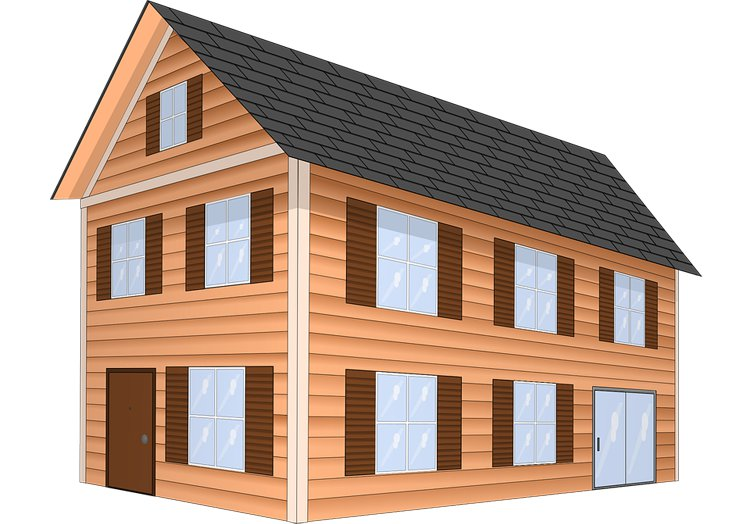# Cube Method Estimating

The cube method estimating is a single rate method of estimating based on the cubic content of a building. Cost per cubic metre method is specific for building projects and aims to overcome the current criticism floor area method that does not take into account possible variations of the storey height. The building volume method became very popular in some European countries like in Germany and Switzerland, where building costs are often expressed in cubic meter prices. The total cost of the project will be given by:

Estimate = Volume X Unit Cost (Cost/m3)

{Volume = External plan area X height X Cost (cost/ m3)}

In order to use the method, the building volume must be first assessed and explicit rules exist in some countries for that purpose. Buildings with distinct types of occupation should have corresponding volumes assessed separately, for example, car park areas, shopping areas and office areas in a commercial building. Specific works like excavations, foundations and external works ought to be assessed separately by using cost comparisons or approximate quantities, for example.

Costs per cubic meter may be difficult to find in countries where the method is not current. Actually, such costs depend on a number of variables, like building types, the proportion of wall area per floor area, quality of finishes and so on.### Cube Method Estimating Example

An office block with a cubic content of 18000m3 has been estimated to cost \$415.00/m3.

Estimate = Cubic content X Rate per cubic metre

Estimate = 18000m3 x \$415.00

Total estimated cost =  \$7,470,000

Calculation of volume is subject to rules of measurement

• Measured from external faces of external walls
• Height of the building is taken from the top of foundation to

For pitched roof

• A point midway between the ceiling and the apex of roof 2/3 where roof space is unoccupied
• A point three-quarters from the ceiling to the apex of the roof where roof space is occupied

For Flat roof

• A point 610mm (2feet) above the roof structure

• Quick method
• Simple math process
• Easy to understand
• Satisfactory on works of a recurring nature.
• Suitable where cubic content of a space or a building directly influences the cost of an element.